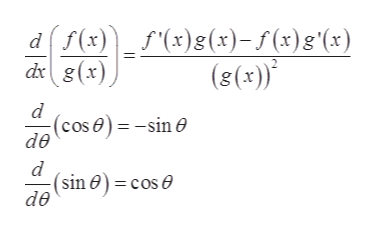2.4Can you show me the steps to solve the problem?Directions: Differentiate.11) sinθ / (1 + cosθ)

Question

2.4

Can you show me the steps to solve the problem?

Directions: Differentiate.

11) sinθ / (1 + cosθ)

Step 1

To determine the first order derivative of the function.

Step 2

Given:

Step 3

Formula Used:

...help_outlineImage Transcriptionclosef(x) (x)8(x)-5 (x)g°(x) dg(x) (s(x)* d (cose)-sin e de d (sin 0) = cos e de fullscreen

Want to see the full answer?

See Solution

Want to see this answer and more?

Our solutions are written by experts, many with advanced degrees, and available 24/7

See Solution
Tagged in

Derivative